# RD Sharma Solutions For Class 7 Maths Chapter 17 Constructions Exercise 17.3

Get the detailed RD Sharma Solutions for Class 7 Maths Exercise 17.3 Chapter 17 Constructions available here. From the exam point of view, subject experts have prepared the solutions in a step by step format in accordance with CBSE syllabus. Students gain knowledge and increase their confidence by following the steps, when practised regularly. This exercise explains SAS triangles and steps involved in constructing them. To know more about constructions students can download RD Sharma Solutions for Class 7 Maths PDF from the links given here.

## Download the PDF of RD Sharma Solutions For Class 7 Maths Chapter 17 – Constructions Exercise 17.3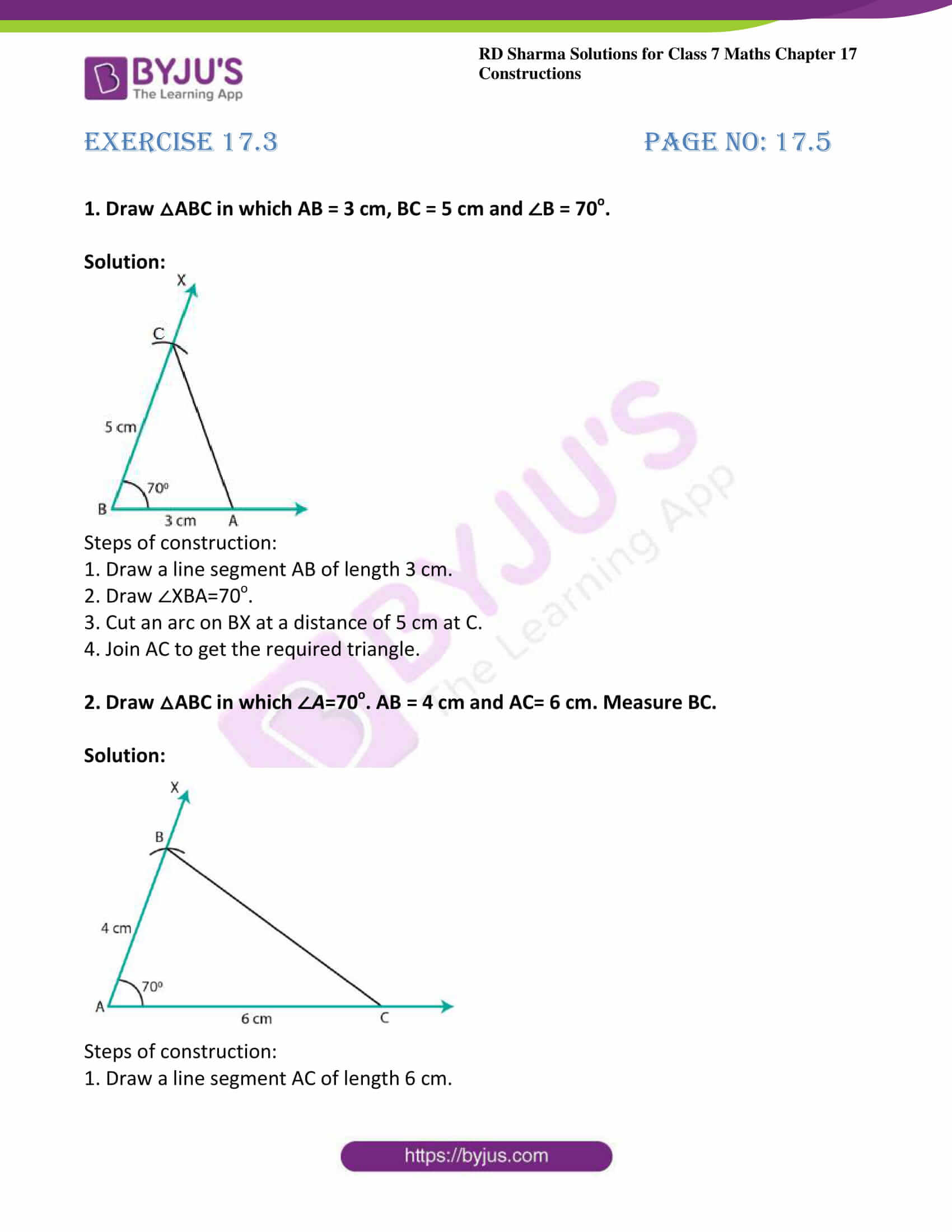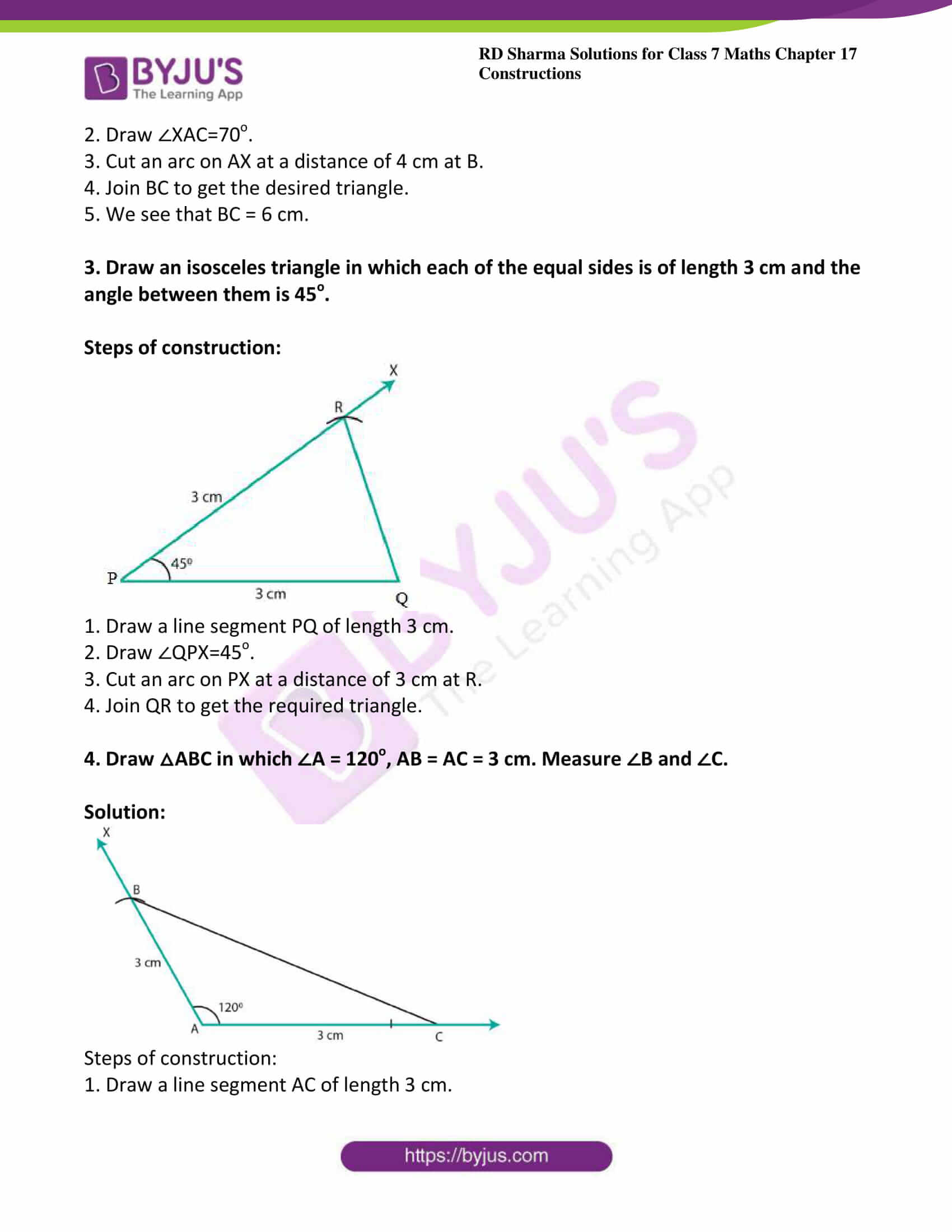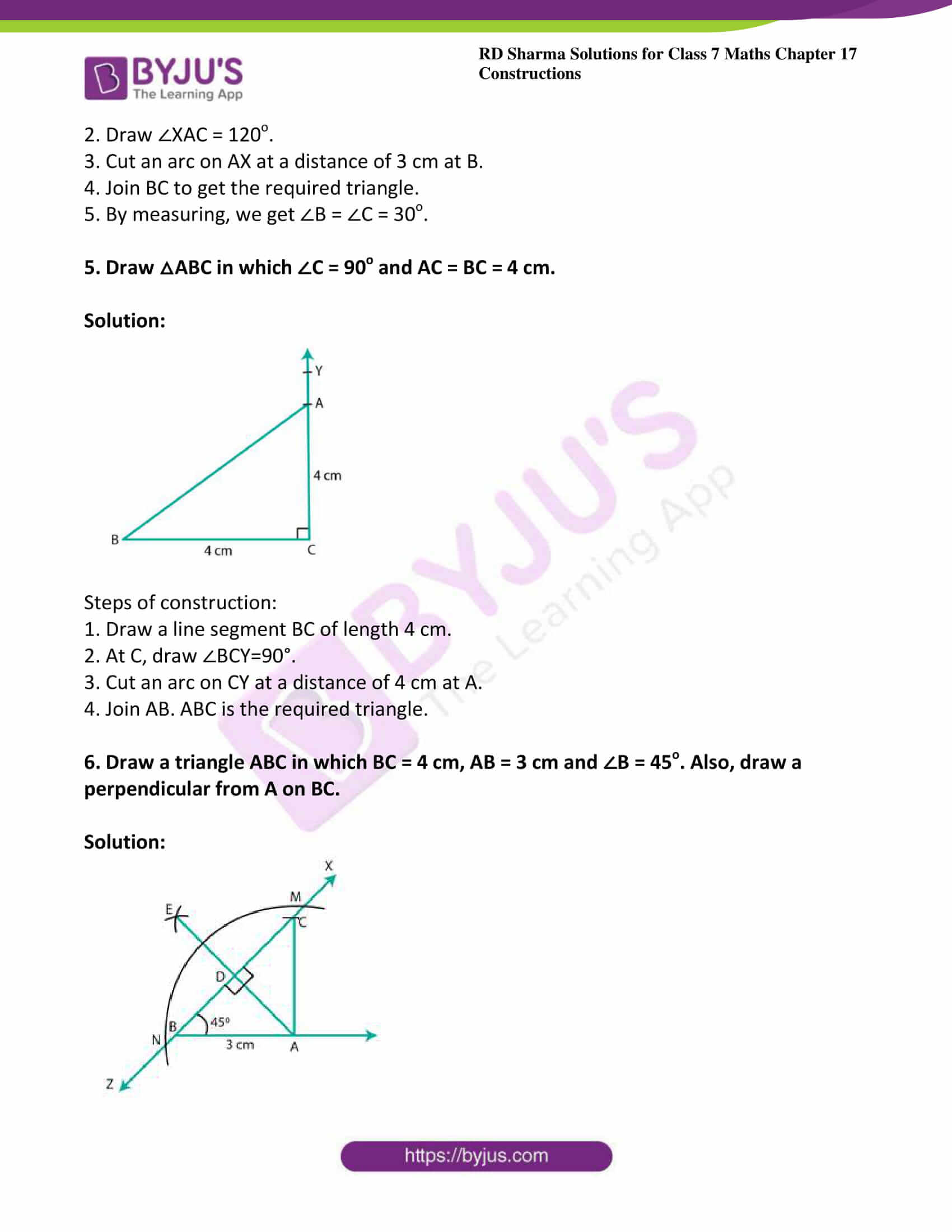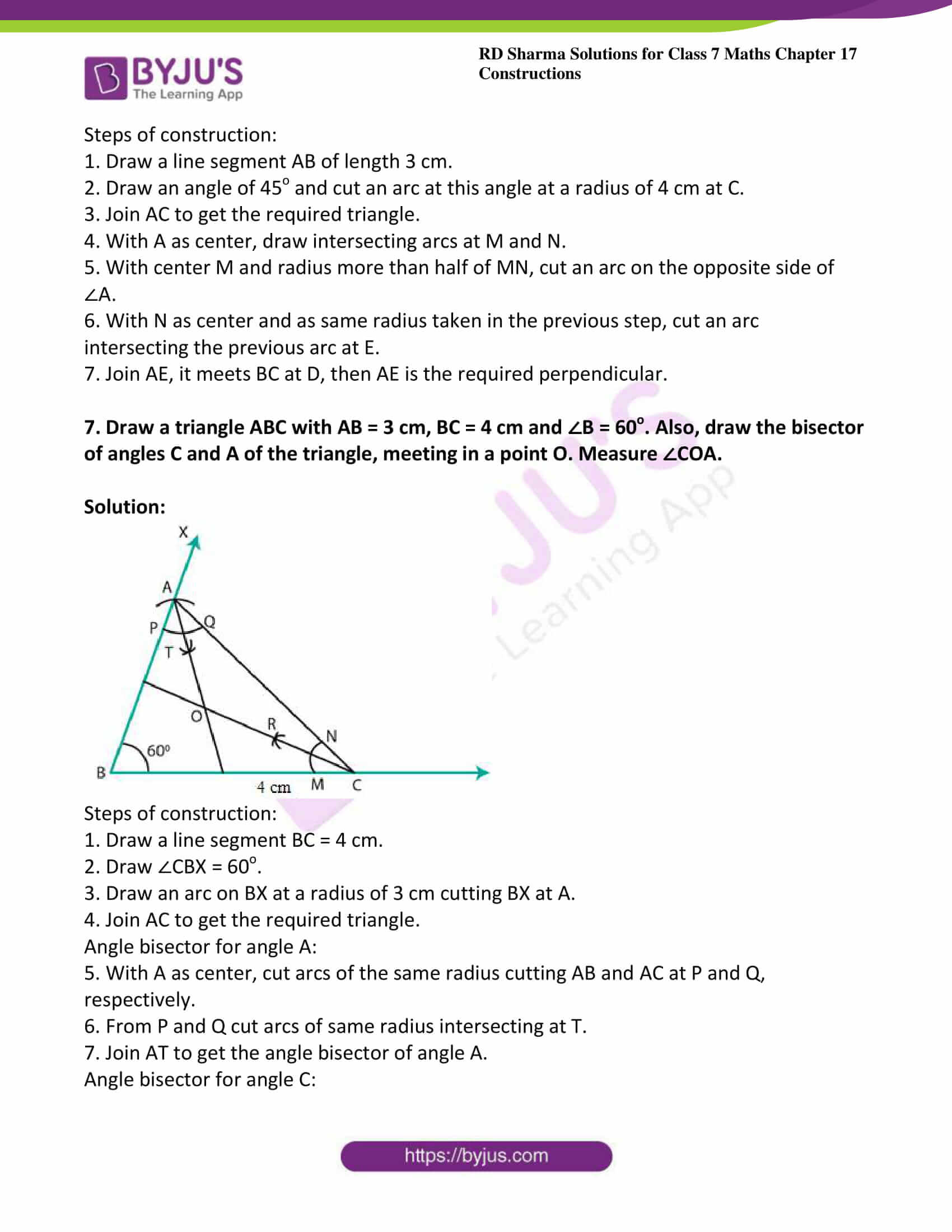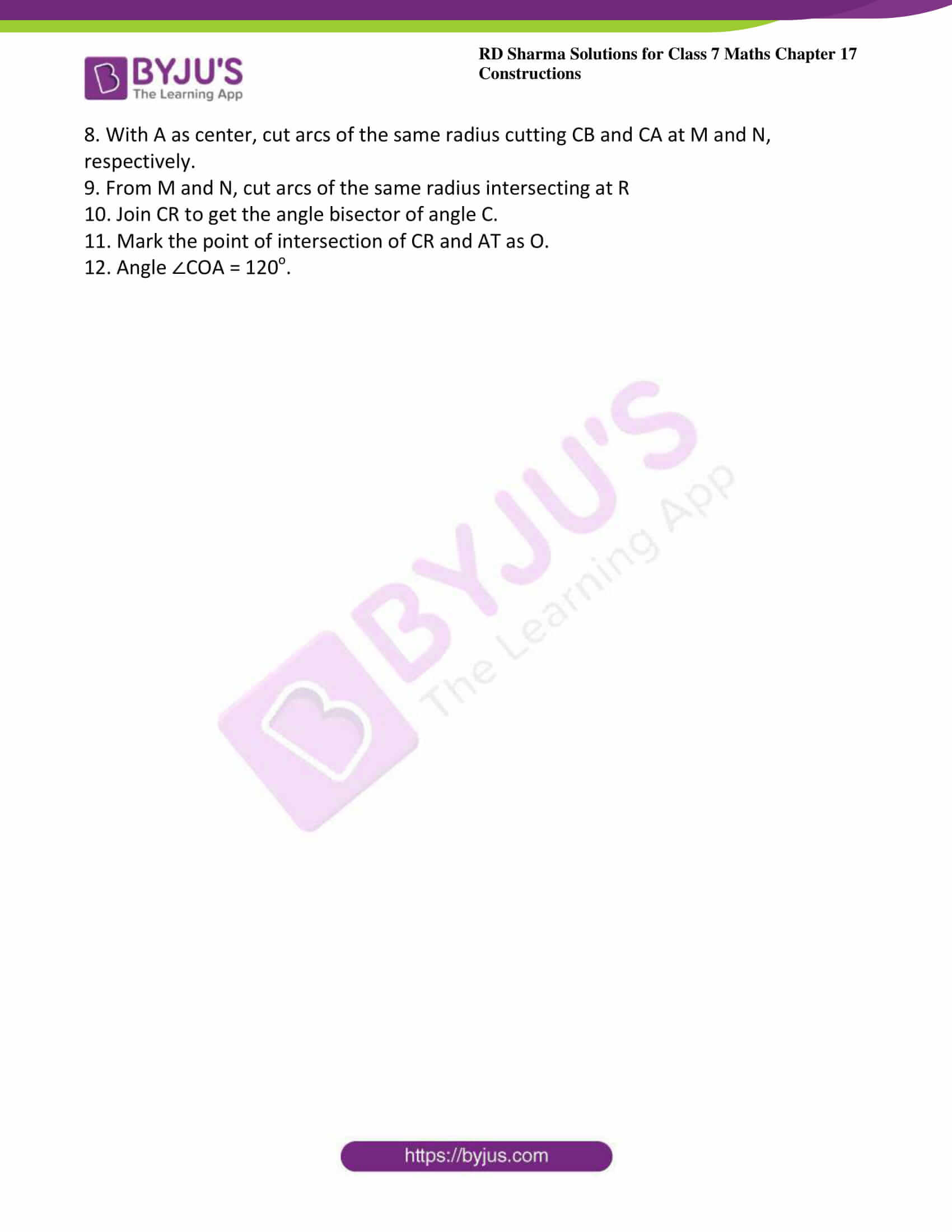### Access answers to Maths RD Sharma Solutions For Class 7 Chapter 17 – Constructions Exercise 17.3

Exercise 17.3 Page No: 17.5

1. Draw △ABC in which AB = 3 cm, BC = 5 cm and ∠B = 70o.

Solution: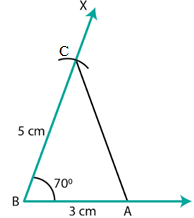Steps of construction:

1. Draw a line segment AB of length 3 cm.

2. Draw ∠XBA=70o.

3. Cut an arc on BX at a distance of 5 cm at C.

4. Join AC to get the required triangle.

2. Draw △ABC in which ∠A=70o. AB = 4 cm and AC= 6 cm. Measure BC.

Solution: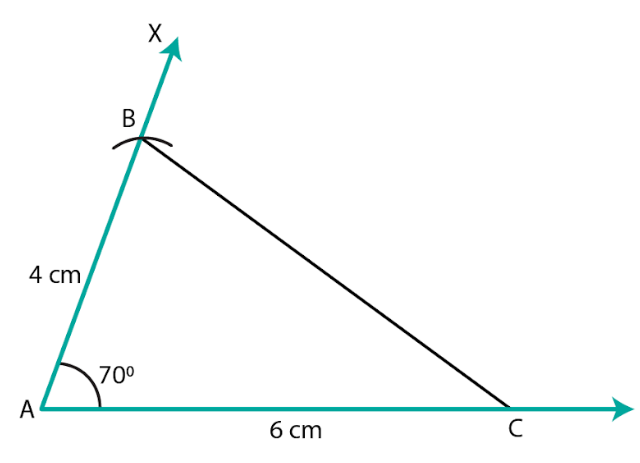Steps of construction:

1. Draw a line segment AC of length 6 cm.

2. Draw ∠XAC=70o.

3. Cut an arc on AX at a distance of 4 cm at B.

4. Join BC to get the desired triangle.

5. We see that BC = 6 cm.

3. Draw an isosceles triangle in which each of the equal sides is of length 3 cm and the angle between them is 45o.

Steps of construction: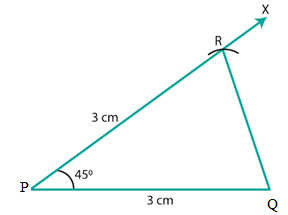1. Draw a line segment PQ of length 3 cm.

2. Draw ∠QPX=45o.

3. Cut an arc on PX at a distance of 3 cm at R.

4. Join QR to get the required triangle.

4. Draw △ABC in which ∠A = 120o, AB = AC = 3 cm. Measure ∠B and ∠C.

Solution: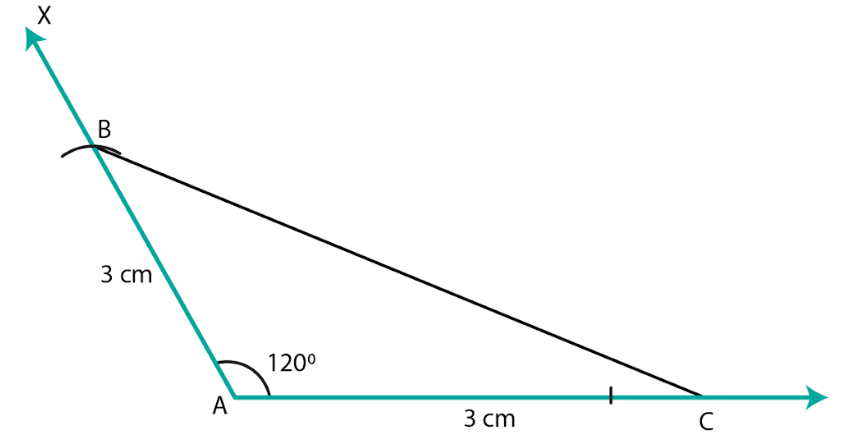Steps of construction:

1. Draw a line segment AC of length 3 cm.

2. Draw ∠XAC = 120o.

3. Cut an arc on AX at a distance of 3 cm at B.

4. Join BC to get the required triangle.

5. By measuring, we get ∠B = ∠C = 30o.

5. Draw △ABC in which ∠C = 90o and AC = BC = 4 cm.

Solution: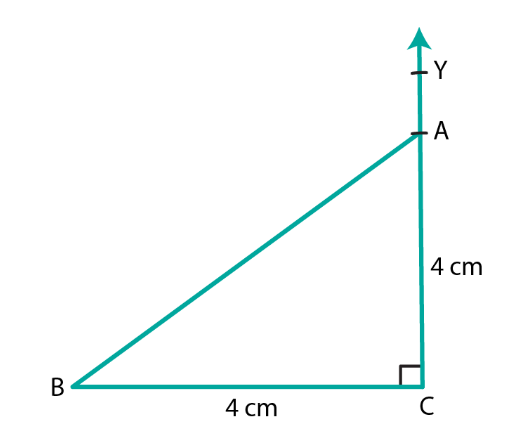Steps of construction:

1. Draw a line segment BC of length 4 cm.

2. At C, draw ∠BCY=90°.

3. Cut an arc on CY at a distance of 4 cm at A.

4. Join AB. ABC is the required triangle.

6. Draw a triangle ABC in which BC = 4 cm, AB = 3 cm and ∠B = 45o. Also, draw a perpendicular from A on BC.

Solution: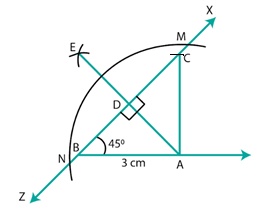Steps of construction:

1. Draw a line segment AB of length 3 cm.

2. Draw an angle of 45o and cut an arc at this angle at a radius of 4 cm at C.

3. Join AC to get the required triangle.

4. With A as center, draw intersecting arcs at M and N.

5. With center M and radius more than half of MN, cut an arc on the opposite side of ∠A.

6. With N as center and as same radius taken in the previous step, cut an arc intersecting the previous arc at E.

7. Join AE, it meets BC at D, then AE is the required perpendicular.

7. Draw a triangle ABC with AB = 3 cm, BC = 4 cm and ∠B = 60o. Also, draw the bisector of angles C and A of the triangle, meeting in a point O. Measure ∠COA.

Solution: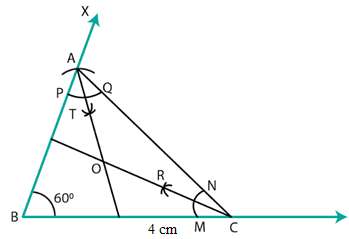Steps of construction:

1. Draw a line segment BC = 4 cm.

2. Draw ∠CBX = 60o.

3. Draw an arc on BX at a radius of 3 cm cutting BX at A.

4. Join AC to get the required triangle.

Angle bisector for angle A:

5. With A as center, cut arcs of the same radius cutting AB and AC at P and Q, respectively.

6. From P and Q cut arcs of same radius intersecting at T.

7. Join AT to get the angle bisector of angle A.

Angle bisector for angle C:

8. With A as center, cut arcs of the same radius cutting CB and CA at M and N, respectively.

9. From M and N, cut arcs of the same radius intersecting at R

10. Join CR to get the angle bisector of angle C.

11. Mark the point of intersection of CR and AT as O.

12. Angle ∠COA = 120o.